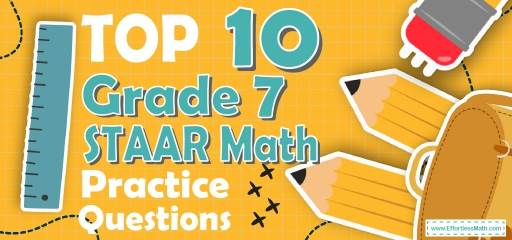# Top 10 7th Grade STAAR Math Practice Questions## 7th Grade STAAR Math Practice Questions

1- What is the area of the shaded region?

A. $$9 π$$ cm$$^2$$

B. $$25 π$$ cm$$^2$$

C. $$39 π$$ cm$$^2$$

D. $$64 π$$ cm$$^2$$

2- A pizza cut into 8 parts. William and his sister Ella ordered two pizzas. William ate $$\frac{1}{4}$$ of his pizza and Ella ate $$\frac{1}{2}$$ of her pizza. What part of the two pizzas was left?

A. $$\frac{1}{2}$$

B. $$\frac{1}{3}$$

C. $$\frac{3}{8}$$

D. $$\frac{5}{8}$$

3- Simplify $$6x^2 y^3 (2x^2 y)^3$$.

A. $$12x^4 y^6$$

B. $$12x^8 y^6$$

C. $$48x^4 y^6$$

D. $$48x^8 y^6$$

4- Right triangle ABC has two legs of lengths 6 cm (AB) and 8 cm (AC). What is the length of the third side (BC)?

A. 4 cm

B. 6 cm

C. 8 cm

D. 10 cm

5- The marked price of a computer is D dollar. Its price decreased by $$20\%$$ in January and later increased by $$10\%$$ in February. What is the final price of the computer in D dollars?

A. 0.80 D

B. 0.88 D

C. 0.90 D

D. 1.20 D

6- $$[6 × (–24) + 8] – (–4) + [4 × 5] ÷ 2 =$$ ?

A. $$-122$$

B. $$-112$$

C. $$-102$$

D. $$-92$$

7- The area of a circle is $$64 π$$. What is the circumference of the circle?

A. $$8 π$$

B. $$16 π$$

C. $$32 π$$

D. $$64 π$$

8- A $40 shirt now selling for$28 is discounted by what percent?

A. $$20 \%$$

B. $$30 \%$$

C. $$40 \%$$

D. $$60 \%$$

9- From last year, the price of gasoline has increased from $1.25 per gallon to$1.75 per gallon. The new price is what percent of the original price?

A. $$72 \%$$

B. $$120 \%$$

C. $$140 \%$$

D. $$160 \%$$

10- If $$40 \%$$ of a class are girls, and $$25 \%$$ of girls play tennis, what fraction of the class play tennis?

A. $$10 \%$$

B. $$15 \%$$

C. $$20 \%$$

D. $$40 \%$$

## Best 7th Grade STAAR Math Prep Resource for 2022

1- C
In this case, to find the area of the shaded region, subtract the area of two circles. (S1: the area of the big circle. S2: the area of a little circle)
Use the area of a circle formula.
S = $$π$$r$$^2$$
$$S_{1} – S_{2}= π( 5 + 3 cm)^2 – π(5 cm)^2$$ ⇒ $$S_{1} – S_{2} = π64 cm^2 – π25 cm^2$$ ⇒ $$S_{1} – S_{2} = 39π cm^2$$

2- D
William ate $$\frac{1}{4}$$ of 8 parts of his pizza that it means 2 parts out of 8 parts ( $$\frac{1}{4}$$ of 8 parts = x ⇒ x = 2) and left 6 parts.
Ella ate $$\frac{1}{2}$$ of 8 parts of her pizza that it means 4 parts out of 8 parts ( $$\frac{1}{2}$$ of 8 parts = x ⇒ x = 4) and left 4 parts.
Therefore, they ate $$(4 + 2)$$ parts out of$$(8 + 8)$$ parts of their pizza and left $$(6 + 4)$$ parts out of $$(8 + 8)$$ parts of their pizza that it means: $$\frac{10}{16}$$
After simplification we have: $$\frac{5}{8}$$

3- D
Simplify.
$$6x^2 y^3 (2x^2 y)^3= 6x^2 y^3 (8x^6 y^3 ) = 48x^8 y^6$$

4- D
Use Pythagorean Theorem: $$a^2 + b^2 = c^2$$
$$62 + 82 = c^2 ⇒ 100 = c^2 ⇒ c = 10$$

5- B
To find the discount, multiply the number by ($$100\%$$ – rate of discount).
Therefore, for the first discount we get:
$$(D) (100\% – 20\%) = (D) (0.80) = 0.80 D$$
For increase of $$10 \%$$:
$$(0.80 D) (100\% + 10\%) = (0.85 D) (1.10) = 0.88 D = 88\% D$$

6- A
Use PEMDAS (order of operation):
$$[6 × (– 24) + 8] – (– 4) + [4 × 5] ÷ 2 = [– 144 + 8] – (– 4) +  ÷ 2 = [– 144 + 8] – (– 4) + 10 =$$
$$[– 136] – (– 4) + 10 = [– 136] + 4 + 10 = – 122$$

7- B
Use the formula of areas of circles.
Area =$$πr^2 ⇒ 64 π = πr^2 ⇒ 64 = r^2 ⇒ r = 8$$
The radius of the circle is 8. Now, use the circumference formula:
Circumference = $$2πr = 2π (8) = 16 π$$

8- B
Use the formula for Percent of Change
$$\frac{New Value-Old Value}{Old Value}× 100 \%$$
$$\frac{28-40}{40}× 100 \% = – 30 \%$$
(negative sign here means that the new price is less than old price).

9- C
The question is this: 1.75 is what percent of 1.25?
Use percent formula:
part =$$\frac{percent}{100}× whole$$
$$\frac{percent}{100}× 1.25$$ ⇒ $$1.75 = \frac{percent ×1.25}{100}⇒175 =$$ percent $$×1.25$$ ⇒ percent $$= \frac{175}{1.25}= 140$$

10- A
Let x be the amount of students in the class.
$$40 \%$$ of x = girls
$$25 \%$$ of girls = tennis player
Input $$40\%$$ of a class instead of girls in second formula. Therefore, $$25\%$$ of $$40\%$$ of a class = tennis player
tennis player = $$10\%$$

## The Best Books to Ace the 7th Grade STAAR MathTest

### What people say about "Top 10 7th Grade STAAR Math Practice Questions - Effortless Math: We Help Students Learn to LOVE Mathematics"?

No one replied yet.

X
30% OFF

Limited time only!

Save Over 30%

SAVE $5 It was$16.99 now it is \$11.99